Function Repository Resource:

# MaximizeOverPermutations

Find the permutation that maximizes a function

Contributed by: Roman Schmied, University of Basel
 ResourceFunction["MaximizeOverPermutations"][f,n] maximizes f numerically with respect to permutations of {1,…,n}.

## Details and Options

ResourceFunction["MaximizeOverPermutations"] takes a Method option that accepts either "Enumerate" or "MonteCarlo".
The number of permutations of n objects scales very quickly with n. The default "Enumerate" method should not be used with n≳12 in order to prevent memory overflow, as the time and space required for this enumeration both scale with n!. A warning is issued, but the user needs to decide whether or not to call for full enumeration of larger permutation spaces.
With the default option setting Method"Enumerate", the maximum is determined by applying the function f to all permutations of the numbers 1 n. The result is guaranteed to be optimal.
With the option setting Method"MonteCarlo", the maximum is determined by a Monte Carlo Metropolis–Hastings stochastic search. There is no guarantee of the result’s optimality. This method makes it possible to search very large permutation spaces efficiently.
With the suboption settings Method{"MonteCarlo",options…}, the Monte Carlo method can be fine-tuned as follows:
The suboption "Iterations"m specifies the number of iterations that the Monte Carlo algorithm performs in the stochastic search. The algorithm's runtime scales linearly with m. The default value is "Iterations"104.
The suboption "AnnealingParameter"β specifies the annealing parameter of the Metropolis–Hastings acceptance/rejection step. Smaller values of β give a wider search; larger values of β tend to restrict the search more toward a monotonically increasing strategy. The default value is "AnnealingParameter"1.
With "AnnealingParameter"{βstart,βend}, the annealing parameter progresses exponentially from βstart at the simulation’s beginning to βend at the end. An increasing annealing parameter is useful to narrow the search progressively during the course of the simulation.
The suboption "StartingPermutation"σ specifies the initial point of the stochastic search in permutation space and must be given as a list σ={s1,s2,,sn} that is a permutation of the numbers 1n. The default value is "StartingPermutation"Automatic, which uses the trivial permutation {1,2,,n} as the starting point.
The Monte Carlo Metropolis–Hastings stochastic search relies on the annealing parameter β, which can be kept uniform or varied exponentially during the simulation. A proposed random step from a permutation σi to a new permutation σi+1 is accepted with probability Max[1,Exp[β(f[σi+1]-f[σi])]]. The annealing parameter β must therefore be chosen in relation to the typically occurring differences in the function f to be maximized. The value of this parameter is crucial for the efficiency of the simulation and must be determined by experimentation.

## Examples

### Basic Examples (3)

Maximize the second value:

 In:=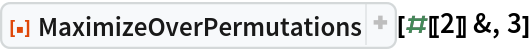Out=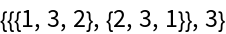Given a function that takes a permutation of the numbers {1,2,3}, determine the maximum function value:

 In:=The result shows that there are two permutations, {1,3,2} and {3,1,2}, that both yield the maximal value f=9:

 In:=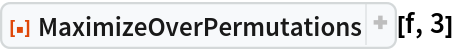Out=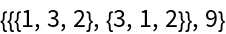Construct a function that takes a permutation of the numbers {1,2,,100} and whose global maximum is known:

 In:=The global maximum of g is given by the permutation that is in the same numerical order as the list x:

 In:=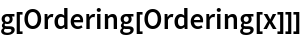Out=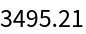Find the maximum of g by a Monte Carlo search though the space of m!≈9×10157 possible permutations:

 In:=Out=This result is not quite optimal, but close. It is much larger than the typical values of g found by applying random permutations directly:

 In:=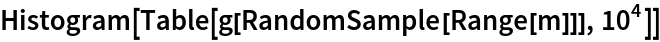Out=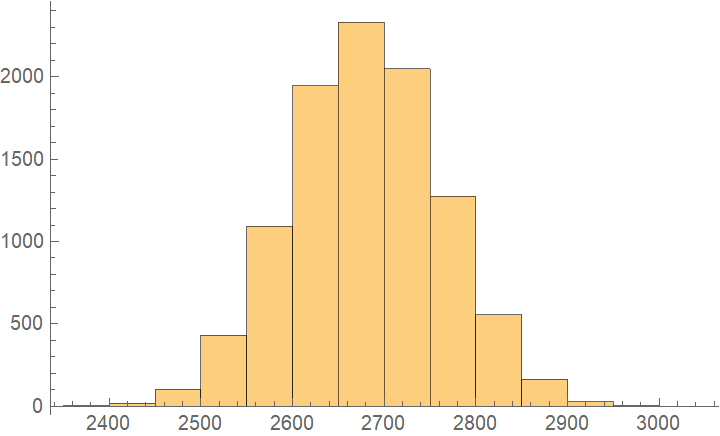### Scope (1)

Minimizing a function f can be achieved by maximizing -f:

 In:=In:=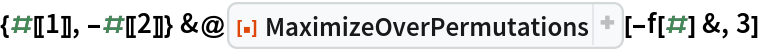Out=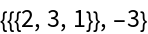### Applications (8)

#### NUG25 (4)

Find the extremum of the NUG25 standard Quadratic Assignment Problem:

 In:=Here is the merit function:

 In:=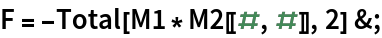The absolute maximum of f is known:

 In:=Out=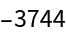Monte Carlo search for the maximum (with no guarantee of maximality):

 In:=Out=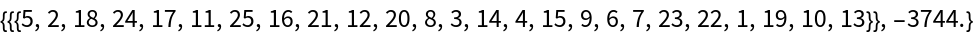#### NUG30 (4)

Find the extremum of the NUG30 standard Quadratic Assignment Problem:

 In:=Here is the merit function:

 In:=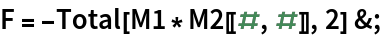The absolute maximum of f is known:

 In:=Out=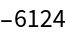Monte Carlo search for the maximum (with no guarantee of maximality):

 In:=Out=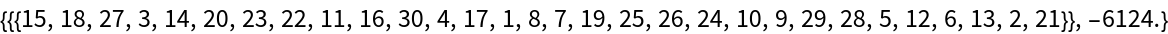Roman Schmied

## Version History

• 1.0.0 – 12 July 2019

## Author Notes

As implemented, the Monte-Carlo algorithm traverses permutation space solely by applying random 2-cycles (that is, by interchanging two random elements at a time). Even though there are n! possible permutations of n objects, any pair of permutations is connected by only n-1 such 2-cycles: permutation space is very well connected and surprisingly small in diameter. For this reason the present implementation does not use larger random cycles to traverse permutation space, and we believe that even highly inhomogeneous maximization problems can be addressed with the present implementation by starting with a sufficiently low annealing parameter β.